# Functions

On the road to the City of Skepticism, I had to pass through the Valley of Ambiguity.

What is a function? The notion of a function is fundamental to mathematics, but like many fundamental aspects of mathematics, its definition was not nailed down until relatively recently. Mathematicians were using functions without thinking all that hard about what functions were! Let's rectify that.

The definition that I prefer to use is the following:

Definition. function from the set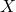to the set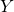is an unambiguous rule which assigns to each element ofan element of.

By unambiguous, I mean that each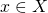has only one element ofassigned to it. This may seem obvious, but in fact there are some interesting mathematical operations that turn out to be a little ambiguous, for example:

Definition. We say thatis a square root of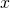if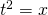.

It's not hard to see that both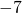and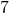are square roots of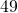, so the phrase the square root of" is actually ambiguous. There are various ways to deal with such ambiguity: for example, we could just content ourselves with the symbol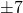, representing the two-element set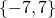, but then the square root of a number isn't a number!. The most straightforward way is just to exclude it by assumption, as I've done with the definition of function I gave above.

To functionize the notion of square root, we usually do the following:

Definition. We say thatis the principal square root of, and write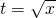, ifand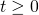.

That is, if there are the two possible choices for square root, we decide always to take the positive one. This notion of the square root" is now unambiguous, i.e. a function.

Let's connect this idea back to our formalism with relations. It's clear thatexpresses a relationship betweenand, so we can hope that our work on relations will help us develop a good definition of function as a kind of relation. For a function fromto, we will want to consider pairs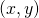, so our relation (let's be traditional and call it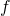) will be a subset of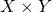.

What would it mean for the rule to be ambiguous? There would be somewith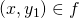and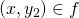, where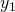andare distinct. That is,being ambiguous means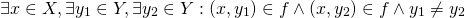Activity. Write a denial of the above logical sentence. This is what it means forto be unambiguous.

Activity. If your denial only includesand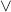, alter it using logical facts so that it contains a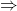.